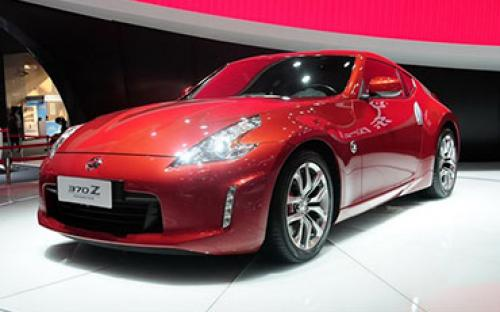### 日产(进口) 日产370Z5 种颜色可选2015款最低售价：52.50 万元起

4265(mm)1848(mm)1318(mm)##### 配置亮点：
• 胎压监测装置

• ISOFIX儿童座椅接口

• 车身稳定控制(ESC/ESP/DSC等)

• 电动天窗

• 定速巡航

• 后倒车雷达

• 真皮座椅

• GPS导航系统

• 氙气大灯

• 后视镜加热

• 提交

日产(进口) 日产370Z 绕车实拍• 日产(进口) 日产370Z 在售车型

排量 车型 厂商指导价 本地最低报价 购车工具
3.7L
3.7L Coupe 7挡手自一体
52.50万
52.50万

3.7L Nismo 7挡手自一体
64.50万
64.50万

日产(进口) 日产370Z 经销商

查看更多 >>

### 日产(进口) 日产370Z 动力加速

日产370Z 0-100公里加速时间分布在 0.0-秒 属于 超跑级

动力级别 加速时间 车型

日产(进口) 日产370Z 视频

日产(进口) 日产370Z 新闻资讯

# 或更名为Z35 日产370Z继任者最新消息

新闻 超过4365次关注

近日，海外媒体报道了关于日产370Z继任者的一些信息，该车未来可能更名为Z35，并搭载来自奔驰的3.0T发动机和一套混合动力系统。据悉，新车预计会先以概念车的身份亮...

# 2014广州车展：日产新款370Z售52.5万元

新闻 超过4664次关注

2014广州车展于11月20日正式拉开帷幕，在本次车展上，日产新款370Z正式上市，新车售价为52.5万元。

# 日产新款370Z Nismo官图发布 更加动感

车系文章 超过3204次关注

日前，日产正式发布了新款370ZNismo车型（海外称为2015款）的官图，新车将在5月15-19日于美国举行的ZDayZ上正式亮相，新车将于今年7月在海外开售。

# 日产新车计划曝光 推新Z系列及GT-R混动版

车系文章 超过2699次关注

日前，据外媒报道，日产将开发新一代Z系列跑车，据悉，新车的外形将会与350Z和370Z有很大的改变，但是具体样式还有待决定，可以确定的是，新的Z系列车身尺寸将更小...

# 日产概念版运动型轿车将亮相底特律车展

车系文章 超过2466次关注

近日，据外媒透露，日产将在底特律车展期间推出一台概念版的运动型轿车，它也将成为日产今后的运动型轿车设计风向标。除了运动型轿车的概念版，日产还会在底特律车...

# 实拍2013款日产370Z 延续运动个性基因

汽车导购 超过4986次关注

在日产品牌的跑车中，相信大家会对GT-R比较熟悉，但此次的文章我们为大家介绍的并非GT-R，而是日产旗下的另一款跑车370Z，这款跑车除了有着个性动感的外观造型外，...

# 试驾日产370Z/GT-R 迟来的爱与梦中的它

试驾评测 超过4661次关注

作为两款早已名声在外的跑车，无论是日产370Z还是GT-R似乎都不用再向人们证明什么，但对于国内消费者而言，久久未能引入的370Z确实需要向这片土地上的人们做出个“...

# 日产370Z改装案例赏析 368马力淑女

车系文章 超过2507次关注

日产370Z已推出多时，近期日产还公布了2014款370ZNISMO版车型。日产370Z在国内的保有量相对较少，虽然外观和动力都很吸引人，但要想得到它需要付出的代价有些高昂（...

猜你喜欢

﻿
• 快速找车
• 选择品牌
• 选择品牌
• A  奥迪
• A  阿斯顿·马丁
• A  阿尔法·罗密欧
• B  宝沃
• B  布加迪
• B  巴博斯
• B  保时捷
• B  宾利
• B  奔驰
• B  宝马
• B  本田
• B  别克
• B  标致
• B  比亚迪
• B  宝骏
• B  北汽制造
• B  北汽新能源
• B  北汽幻速
• B  北汽威旺
• B  北京汽车
• B  奔腾
• B  北汽绅宝
• C  长安
• C  长安商用
• C  长城
• C  昌河
• D  大众
• D  道奇
• D  DS
• D  东南
• D  东风风神
• D  东风风行
• D  东风小康
• D  东风风度
• D  东风
• F  福特
• F  丰田
• F  菲亚特
• F  法拉利
• F  福田
• F  福迪
• F  福汽启腾
• G  观致
• G  广汽传祺
• G  广汽吉奥
• G  GMC
• H  红旗
• H  汉腾汽车
• H  哈弗
• H  哈飞
• H  海格
• H  海马
• H  华颂
• H  黄海
• H  华泰
• H  恒天
• J  吉利汽车
• J  捷豹
• J  Jeep
• J  江淮
• J  江铃
• J  金杯
• J  九龙
• J  金旅
• K  凯翼
• K  凯迪拉克
• K  克莱斯勒
• K  科尼塞克
• K  卡威
• K  开瑞
• L  路虎
• L  林肯
• L  劳斯莱斯
• L  兰博基尼
• L  雷克萨斯
• L  铃木
• L  雷诺
• L  理念
• L  力帆
• L  莲花汽车
• L  猎豹
• L  路特斯
• L  陆风
• M  马自达
• M  MG
• M  MINI
• M  玛莎拉蒂
• M  摩根
• M  迈凯轮
• N  纳智捷
• O  欧宝
• O  讴歌
• O  欧朗
• Q  奇瑞
• Q  起亚
• Q  启辰
• R  日产
• R  荣威
• R  瑞麒
• S  三菱
• S  斯威汽车
• S  萨博
• S  smart
• S  斯柯达
• S  斯巴鲁
• S  思铭
• S  双龙
• S  上汽大通
• S  双环
• T  特斯拉
• T  腾势
• W  沃尔沃
• W  五菱汽车
• W  五十铃
• W  威兹曼
• W  威麟
• X  现代
• X  雪佛兰
• X  雪铁龙
• X  西雅特
• Y  一汽
• Y  英菲尼迪
• Y  英致
• Y  依维柯
• Y  野马汽车
• Y  永源
• Z  众泰
• Z  中华
• Z  中兴
• Z  知豆
• 选择车系
• 选择车系
• 车型对比
• 选择品牌
• 选择品牌
• A  奥迪
• A  阿斯顿·马丁
• A  阿尔法·罗密欧
• B  宝沃
• B  布加迪
• B  巴博斯
• B  保时捷
• B  宾利
• B  奔驰
• B  宝马
• B  本田
• B  别克
• B  标致
• B  比亚迪
• B  宝骏
• B  北汽制造
• B  北汽新能源
• B  北汽幻速
• B  北汽威旺
• B  北京汽车
• B  奔腾
• B  北汽绅宝
• C  长安
• C  长安商用
• C  长城
• C  昌河
• D  大众
• D  道奇
• D  DS
• D  东南
• D  东风风神
• D  东风风行
• D  东风小康
• D  东风风度
• D  东风
• F  福特
• F  丰田
• F  菲亚特
• F  法拉利
• F  福田
• F  福迪
• F  福汽启腾
• G  观致
• G  广汽传祺
• G  广汽吉奥
• G  GMC
• H  红旗
• H  汉腾汽车
• H  哈弗
• H  哈飞
• H  海格
• H  海马
• H  华颂
• H  黄海
• H  华泰
• H  恒天
• J  吉利汽车
• J  捷豹
• J  Jeep
• J  江淮
• J  江铃
• J  金杯
• J  九龙
• J  金旅
• K  凯翼
• K  凯迪拉克
• K  克莱斯勒
• K  科尼塞克
• K  卡威
• K  开瑞
• L  路虎
• L  林肯
• L  劳斯莱斯
• L  兰博基尼
• L  雷克萨斯
• L  铃木
• L  雷诺
• L  理念
• L  力帆
• L  莲花汽车
• L  猎豹
• L  路特斯
• L  陆风
• M  马自达
• M  MG
• M  MINI
• M  玛莎拉蒂
• M  摩根
• M  迈凯轮
• N  纳智捷
• O  欧宝
• O  讴歌
• O  欧朗
• Q  奇瑞
• Q  起亚
• Q  启辰
• R  日产
• R  荣威
• R  瑞麒
• S  三菱
• S  斯威汽车
• S  萨博
• S  smart
• S  斯柯达
• S  斯巴鲁
• S  思铭
• S  双龙
• S  上汽大通
• S  双环
• T  特斯拉
• T  腾势
• W  沃尔沃
• W  五菱汽车
• W  五十铃
• W  威兹曼
• W  威麟
• X  现代
• X  雪佛兰
• X  雪铁龙
• X  西雅特
• Y  一汽
• Y  英菲尼迪
• Y  英致
• Y  依维柯
• Y  野马汽车
• Y  永源
• Z  众泰
• Z  中华
• Z  中兴
• Z  知豆
• 选择车系
• 选择车系
• 选择车型
• 选择车型
• 意见反馈